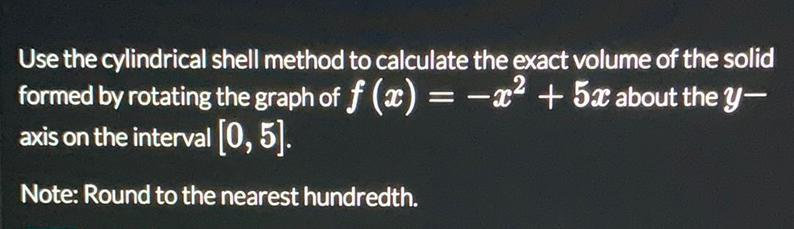Question:

# Use the cylindrical shell method to calculate the exact volume of the solid formed by rotating the graph of f (x) = -x2 + 5x aboUse the cylindrical shell method to calculate the exact volume of the solid formed by rotating the graph of f (x) = -x2 + 5x about the y– axis on the interval (0,5). Note: Round to the nearest hundredth.